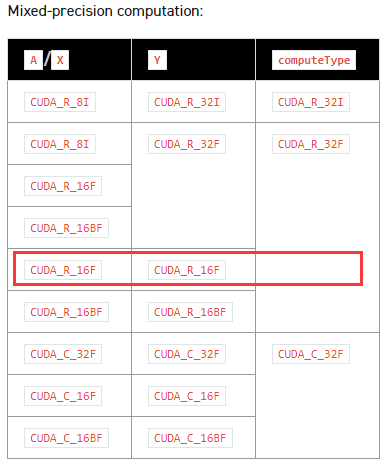# How does parameter computeType affect the computation?

Hi,
I’m a little confused about how the parameter `computeType` affects the calculation.

For example, in cusparseSpMV, the types of A, X and Y are 16f and `computeType` is `CUDA_R_32F`. Does it mean that in the computation, the types of A, X and Y will be converted to f32 first, then they will be calculated, and finally the result Y will be converted to f16?Thank you.

This would be the typical, original tensor core calculation. The calculation is a 16-bit float by a 16-bit float, yielding a 32-bit float result. Corresponding results (within a single tensor core op) are accumulated in 32-bit float. The accumulated result is converted back to 16-bit float upon storage, i.e. on completion of the underlying sass tensor core operation. You can refer to diagrams 7 and 8 here, where the only deviation from diagram 8 in this case is that the FP32 result gets converted to FP16 at the point of storage of that result.

2 Likes

This topic was automatically closed 14 days after the last reply. New replies are no longer allowed.